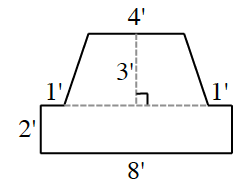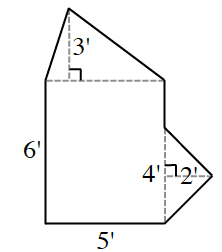### Home > INT2 > Chapter 2 > Lesson 2.1.2 > Problem2-18

2-18.

​​ Determine the area of each of the following figures. Assume that all angles that look like right angles are right angles.

1.Notice there is a trapezoid and rectangle in the diagram.

$A=31$ sq. ft.

1.Notice the dashed lines at the base of each triangle. Try to find the area of each shape and add them together to find the total area.

$A=41.5$ sq. ft.